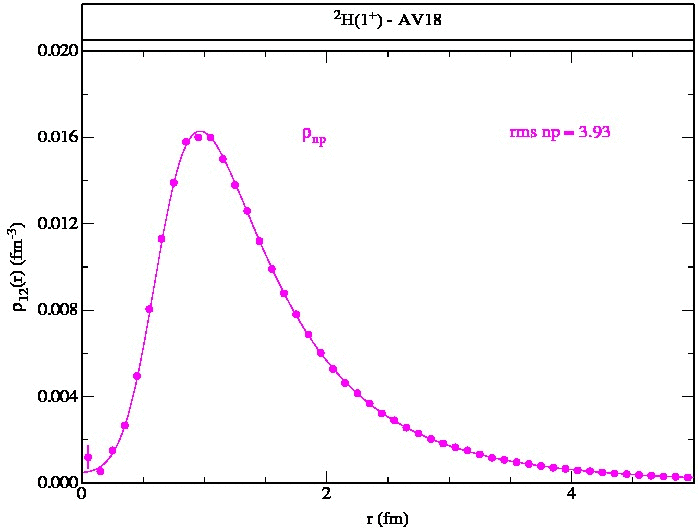# Two-Nucleon Densities

## Single-Nucleon DensitiesThis web page presents two-nucleon densities calculated for a variety of nuclei in the range A=2-10 with some preliminary results for A=11,12. Corresponding single-nucleon densities can be found here. These are from variational Monte Carlo calculations (VMC) using (unless otherwise noted) the Argonne v18 two-nucleon and Urbana X three-nucleon potentials (AV18+UX). (Urbana X is intermediate between the Urbana IX and Illinois-7 models; it has the form of UIX supplemented with a two-pion S-wave piece, while the strengths of its terms are taken from the IL7 model. It does NOT have the three-pion-ring term of IL7.)

These VMC wave functions are the starting trial functions for a number of recent Green's function Monte Carlo (GFMC) calculations:
Brida, et al., Phys. Rev. C 84, 024319 (2011);
McCutchan, et al., Phys. Rev. C 86, 024315 (2012);
Pastore, et al., Phys. Rev. C 87, 035503 (2013);
Pastore, et al., Phys. Rev. C 90, 024321 (2014).

More details of the wave function construction can be found in
Wiringa, Phys. Rev. C 43, 1585 (1991) for A=3,4;
Pudliner, et al., Phys. Rev. C 56, 1720 (1997) for A=6,7;
Wiringa, et al., Phys. Rev. C 62, 014001 (2000) for A=8;
Pieper, et al., Phys. Rev. C 70, 044310 (2002) for A=9,10.

The largest nuclei are evaluated using the cluster VMC (CVMC) method.

The CVMC method is described in
Pieper, et al., Phys. Rev. C 46, 1741 (1992) for A=16 with AV1 4+UVII
Lonardoni, et al., arXiv:1705.04337 for A=16,40 with AV18+UIX.

The results are generated as distributions for proton-proton, neutron-proton and neutron-neutron (where different). The densities are for the same wave functions used in generating the two-nucleon momentum distributions given here.

Following are figures and files that tabulate the two-nucleon densities to give an overall view of their shapes. The normalization is chosen such that:

Z(Z-1)/2 = ∫ d3r ρpp(r)
NZ = ∫ d3r ρnp(r)
N(N-1)/2 = ∫ d3r ρnn(r)

Where pp and nn densities are the same, as in T=0 nuclei, we give only one set.
 2H(1+) Figure Table 3H(1/2+) Figure Table 3He(1/2+) Figure Table 4He(0+) Figure Table 4He(0+) AV18 Figure Table 5H(1/2+) Figure Table 6He(0+) Figure Table 6Li(1+) Figure Table 6Be(0+) Figure Table 7Li(3/2-) Figure Table 7Be(3/2-) Figure Table 8He(0+) Figure Table 8Li(2+) Figure Table 8Be(0+) Figure Table 8B(2+) Figure Table 8C(0+) Figure Table 9Li(3/2-) Figure Table 9Be(3/2-) Figure Table 9C(3/2-) Figure Table 10Be(0+) updated Figure Table 10B(3+) updated Figure Table 11Li(3/2-) Figure Table 11B(3/2-) updated02-Aug-18 Figure Table 12Be(0+) updated18-Aug-18 Figure Table 12C(0+) updated02-Jun-18 Figure Table 16O(0+) CVMC AV18+UIX Figure Table 16O(0+) CVMC AV18 Figure Table 40Ca(0+) CVMC AV18+UIX Figure Table 40Ca(0+) CVMC AV18 Figure Table

Robert B. Wiringa
Last update Aug 20, 2018